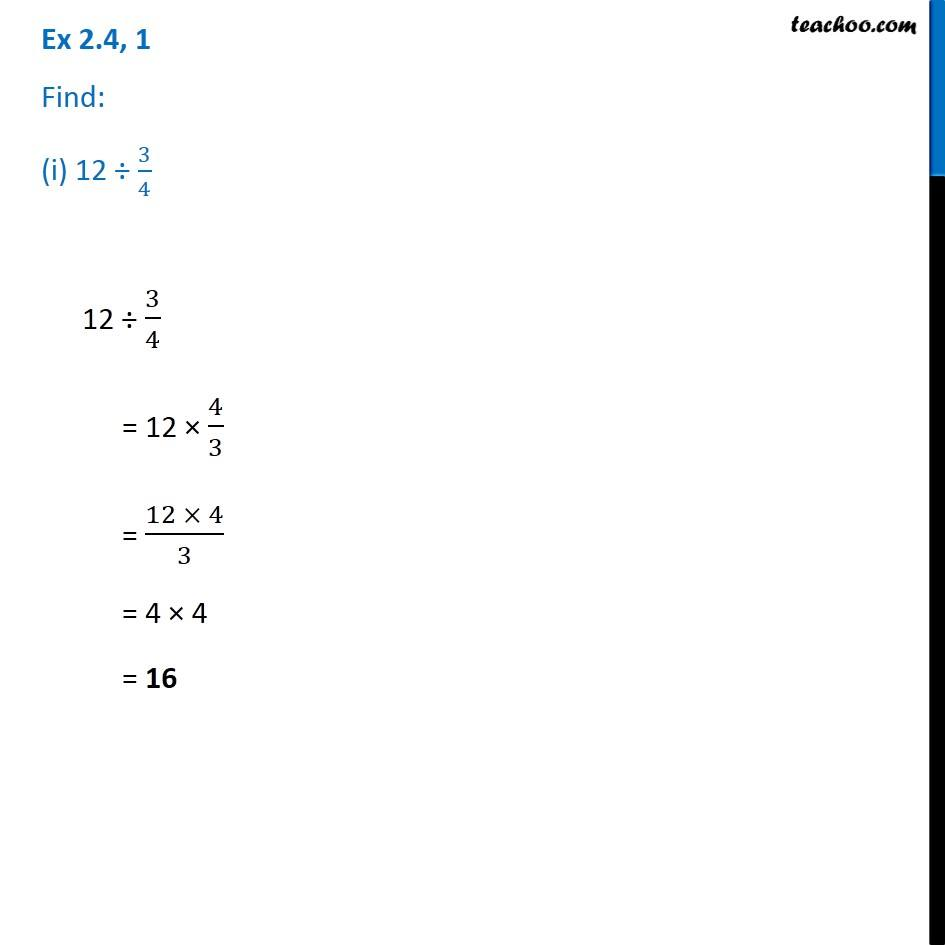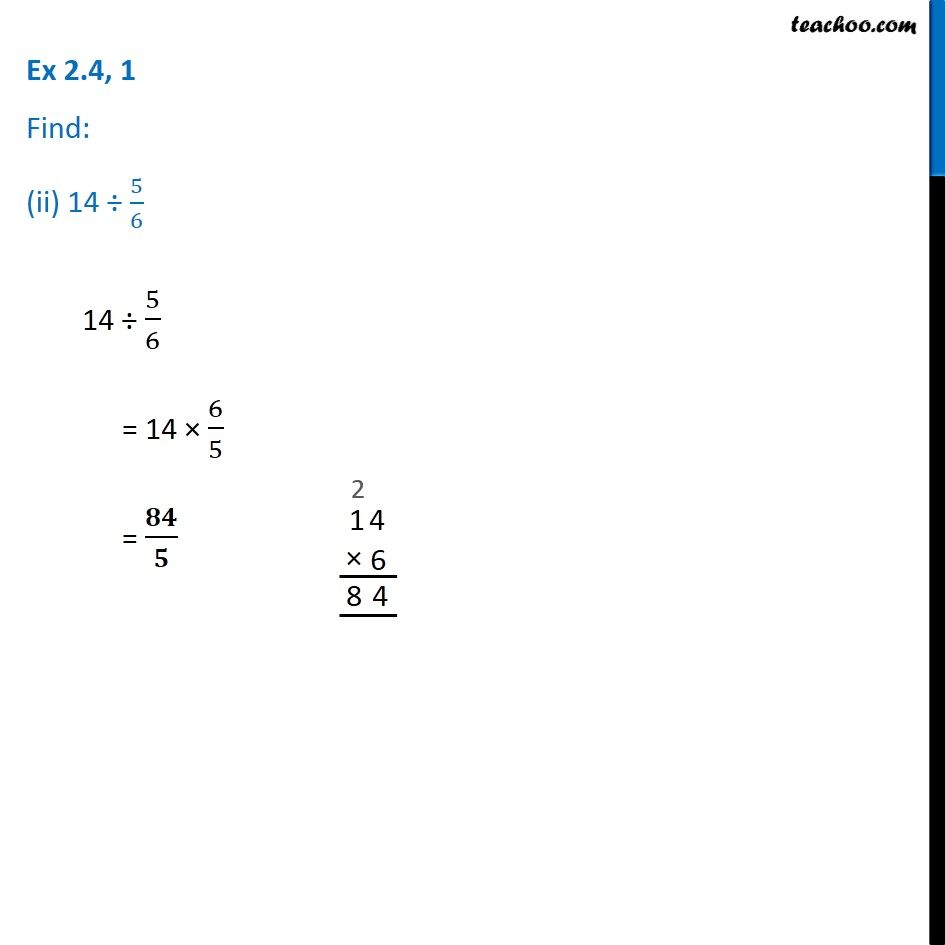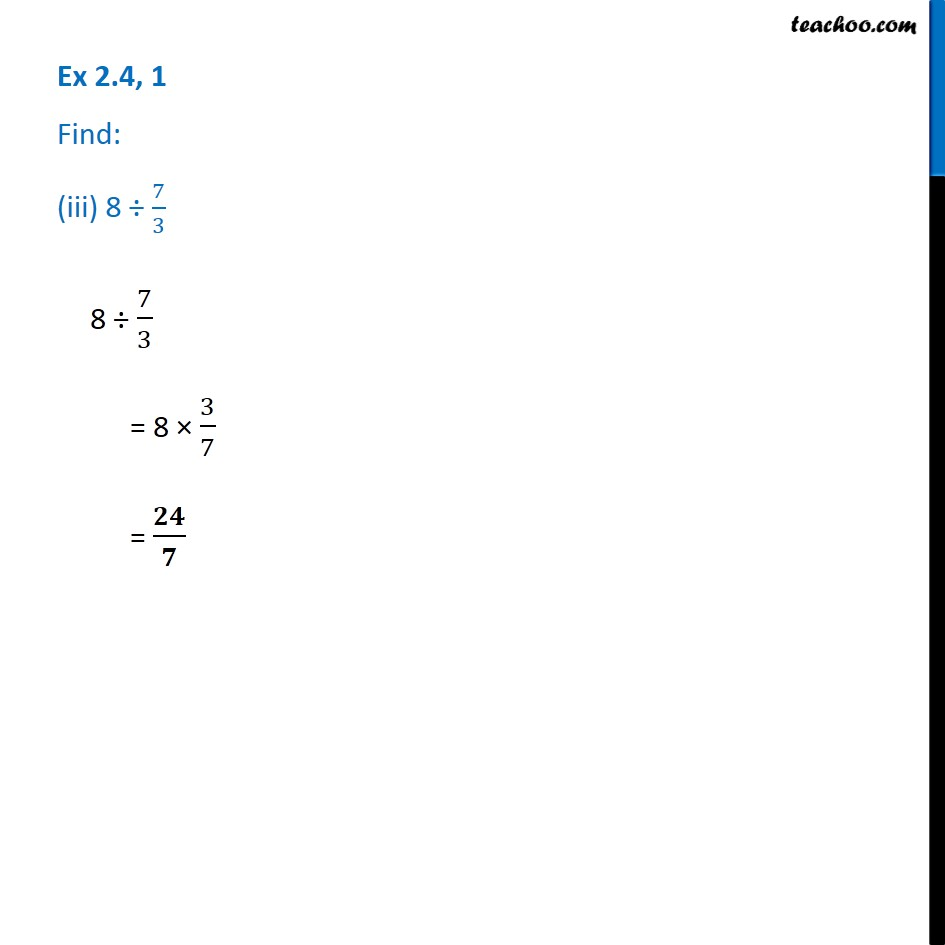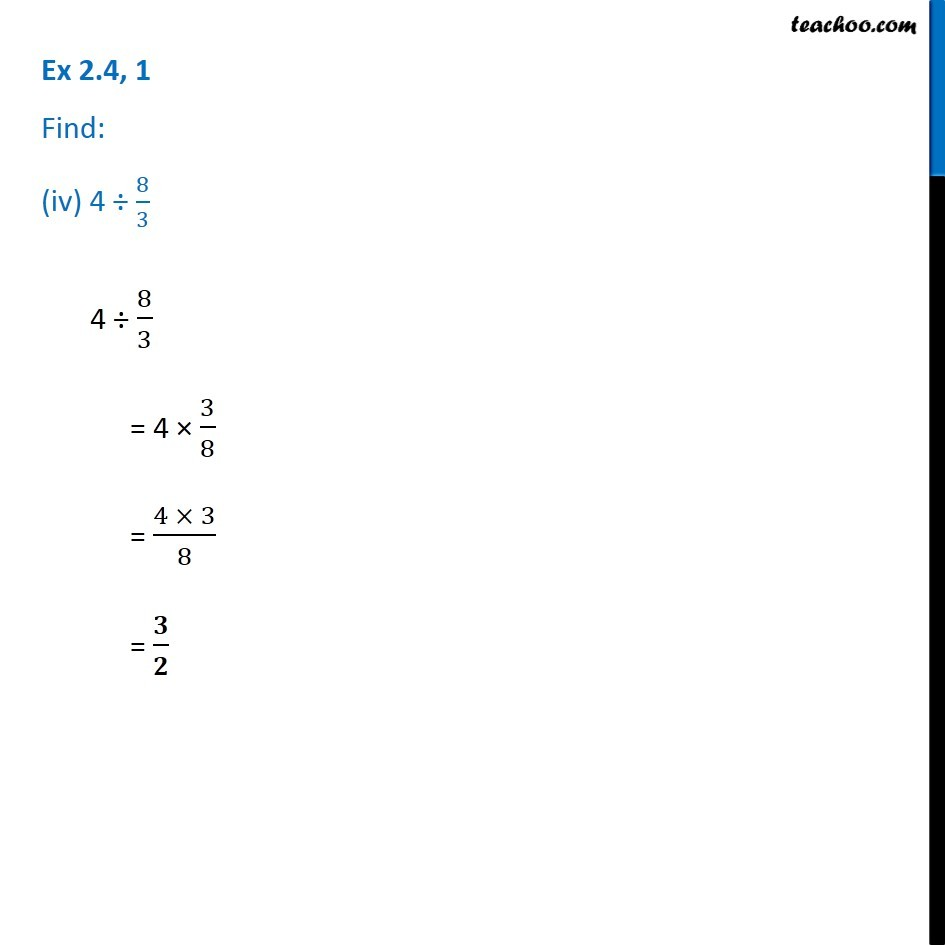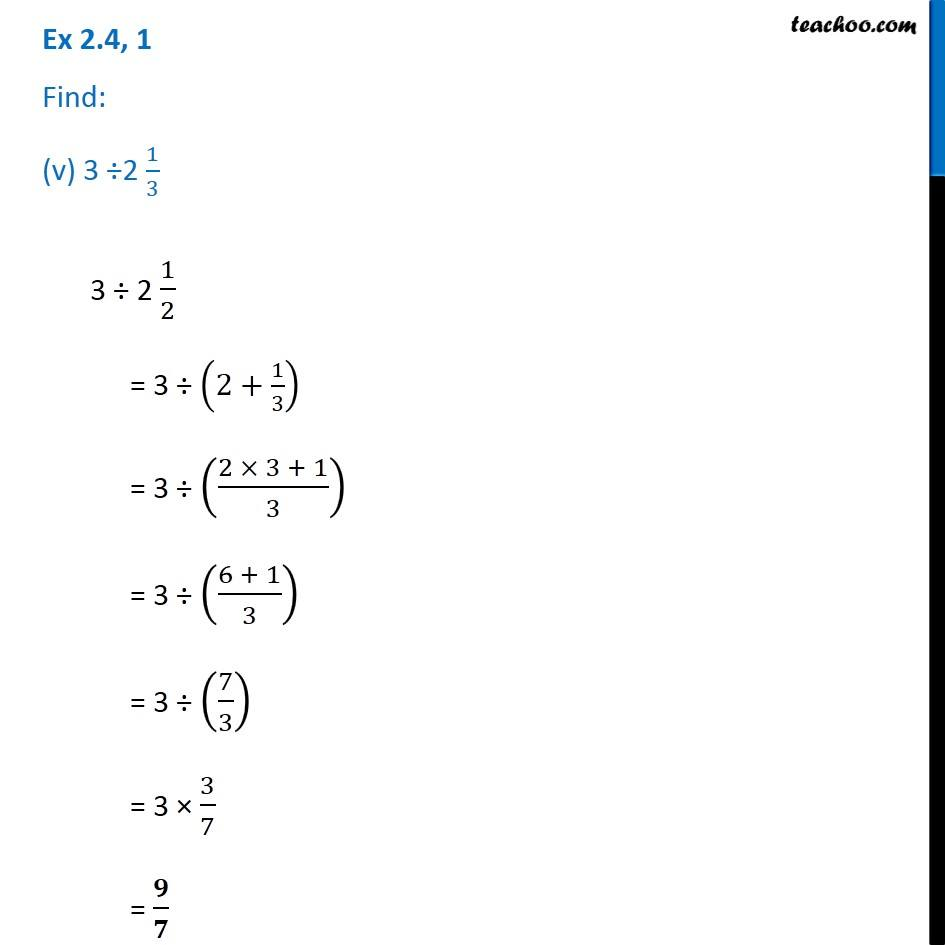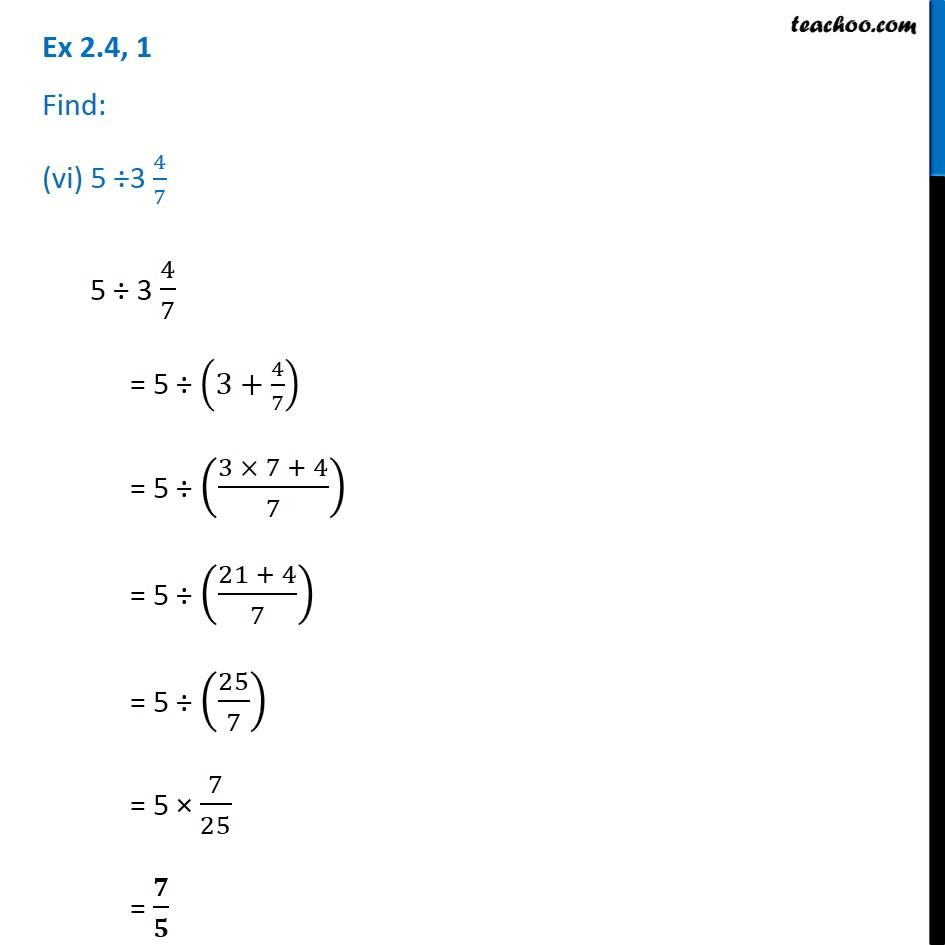1. Chapter 2 Class 7 Fractions and Decimals
2. Serial order wise
3. Ex 2.4

Transcript

Ex 2.4, 1 Find: (i) 12 ÷ 3/4 12 ÷ 3/4 = 12 × 4/3 = (12 × 4)/3 = 4 × 4 = 16 Ex 2.4, 1 Find: (ii) 14 ÷ 5/614 ÷ 5/6 = 14 × 6/5 = 𝟖𝟒/𝟓 Ex 2.4, 1 Find: (iii) 8 ÷ 7/3 8 ÷ 7/3 = 8 × 3/7 = 𝟐𝟒/𝟕 Ex 2.4, 1 Find: (iv) 4 ÷ 8/34 ÷ 8/3 = 4 × 3/8 = (4 × 3)/8 = 𝟑/𝟐 Ex 2.4, 1 Find: (v) 3 ÷2 1/33 ÷ 2 1/2 = 3 ÷ (2+1/3) = 3 ÷ ((2 × 3 + 1)/3) = 3 ÷ ((6 + 1)/3) = 3 ÷ (7/3) = 3 × 3/7 = 𝟗/𝟕 Ex 2.4, 1 Find: (vi) 5 ÷3 4/7 5 ÷ 3 4/7 = 5 ÷ (3+4/7) = 5 ÷ ((3 × 7 + 4)/7) = 5 ÷ ((21 + 4)/7) = 5 ÷ (25/7) = 5 × 7/25 = 𝟕/𝟓

Ex 2.4